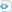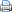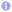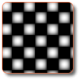This (->) is not only a matter of style.

Quote:

Original post by Saruman

Quote:

 Original post by caldiar What I’m doing wouldn’t happen to be using that hidden this pointer I keep reading about would it?

That is exactly what you are doing by using this->function2() although you don’t need it at all. In C++ this-> is implied when calling member functions.

There is really zero reason you would ever do that in front of a function, but some people use this-> before member variables in order to avoid code warts. (i.e. instead of naming member variables something like: m_myVariable they will use this->myVariable)

Using this-> is actually a good practice if you write templates.

Consider the following example:

```Code block#include <iostream>

void vfun() {
std::cout << "::vfun()n";
}

template <typename T> struct X {
virtual void vfun() const { std::cout << "X::vfun()n"; }
};

template <typename T> struct Y : public X<T> {
void fun () { vfun(); }
};

template <typename T> struct Z : Y<T> {
virtual void vfun() const { std::cout << "Z::vfun()n"; }
};

int main () {
Z<int> z;
z.fun();
std::cout << std::flush;
}```

Remember that templates are parsed two times: One time the template definition itself is parsed, so errors in the template itself can be found, and another time upon instantiation, so errors resulting from specialisations can be found (early C++ compilers implemented templates more like ordinary #define macros; but we know how hard finding bugs can be that way). Also, only during the second phase, “templated” base classes can be looked up.

At first, a global function vfun() is defined. Nothing special. Then, a class template X<T> is defined. X defines a virtual function “vfun()”.

Another class template Y<T> is then defined, which derives from X<Y>, but it does not re-implement the virtual function X::vfun(). No problem yet. Y<T> also defines a member function, named fun(), which itself calls vfun(). (There’s a third class template, struct Z<T>, but we ignore it for now.)

Here’s struct Y again:

```Code blocktemplate <typename T> struct Y : public X<T> {
void fun () { vfun(); }
};```

The problem now is, that the call to vfun() is unqualified (no ::vfun() or Y<X>::vfun(), just the pure function name). Furthermore, the arguments you pass to it don’t depend on a template parameter (in this example, we are actually passing no argument at all). Thus, the call doesn’t qualify for Argument Dependent Lookup (ADL).

You would now think, “why does the compiler not just lookup the baseclass?”. The answer lies in possible side effects due to explicit or partial specialisations (which can legally occur everywhere in the code), which basically means that e.g. a template specialisation X<bool> could be defined in a completely different way than X<float>, e.g. X<bool> could define “enum { pipapo = 0 };”, whereas X<float> could  define pipapo as “typedef X pipapo;”.

So, what remains is to use ordinary lookup of non-dependent names only, which is approximately the type of lookup you would have in a C program,  because during the second parse, only ADL and lookup of dependent names occur.

Explicitly qualifying the call to vfun()

If there wouldn’t be a global vfun() (just try yourself), standards compliant compilation should trigger an error about a call to an undeclared function. But with the global vfun(), the compiler happily finds it unambiguously using ordinary lookup. Thus, instead of calling the base classes’ virtual member function “vfun()”, Y<T>::fun() will call the global one.

A solution to this is to qualify the name:

```Code blocktemplate <typename T> struct Y : public X<T> {
void fun () { X<T>::vfun(); }
};```

Now, the correct function gets called, because we made the call dependent upon a template parameter, so that name-lookup is delayed until the second phase.

We just inhibited the virtual function call mechanism

But still, we have a problem if another class derives from Y<> and overrides vfun(). Here, our previously ignored struct Z<T> comes into play:

```Code blocktemplate <typename T> struct Z : Y<T> {
virtual void vfun() const { std::cout << "Z::vfun()n"; }
};```

Note that Z<T> also derives fun() from Y<T>, our previous “problem” function. But in “Y<T>::fun()”, we qualified the call to vfun() with X<T>::vfun(), which unfortunately means that we inhibited virtual function calling rules, and have fallen back to a non-virtual call. So, if we now call Z<T>::vfun() through a pointer or reference to struct X, we really call X<T>::vfun(), and not the overriden version in Z<T>.

While this might have been intentional, what if you really want to call the most derived vfun() inside fun(), instead of calling the least derived version? How can me make the virtual call dependent on a template parameter so that lookup can be delayed to the second phase of template parsing?, without interfering with any derivation rules?

this-> is the solution

Consider that the this-> pointer itself is a dependent name inside a class template, as its type is only completely determined once the template is intantiated (-> lookup delayed to second phase). So the simple solution to our not too obvious problem is, when writing a template, to use this-> whenever possible (*).

Here is our modified example:

```Code blocktemplate <typename T> struct Y : public X<T> {
void fun () { this->vfun(); }
};```

So, actually, using “this->” whenever possible is good style when writing templates. It is not needed in case of an explicit specialisation (**), because an explicit specialisation is basically the same as an ordinary non-template class or function, where each entity can be looked up upon the first parse (in an explicit specialisation, this-> is non-dependent, so we could not even direct the lookup until a second phase as all non-dependent names are only looked up in the first phase; despite that no second phase exists for explicit specialisations (***)).

Footnotes

(*) Except, of course, in the case where we are really interested in a very specific entity, like the global vfun() in our example.

(**) If you would write the following specialisation, this-> can be ommitted, as the compiler can fully instantiate X<int> and Y<int>, and thus find the virtual member function vfun().

```Code blocktemplate <> struct Y<int> : X<int> {
void fun () { vfun(); } // Will call the virtual
// function, not the global one.
};```

(***) Though a partial specialisation still contains dependent names, and all of the said still applies.

Appendix

In an answer to Hummm below, I’ve mashed up the following snippet for you to play with. It has some explanations; just don’t forget about two-phase lookup:

```Code block#include <iostream>
void vfun() {
std::cout << "::vfun()\n";
}

template <typename T> struct X {
virtual void vfun() const { std::cout << "X::vfun()\n"; }
};

template <typename T> struct Y : public X<T> {
// During compilation, only the global vfun()
// is visible, so this will call ::vfun().
void fun0 () { vfun(); }

// This will explitily call X::vfun()
// and inhibit virtual function dispatch.
void fun1 () { X<T>::vfun(); }

// Qualifying the call with this makes the call
// to vfun() "dependent", as the type of 'this'
// depends on X<T>, which is not yet known.
// Thus, the compiler will postpone
// name-lookup until Phase 2 of template parsing.
void fun2 () { this->vfun(); }
};

template <typename T> struct Z : Y<T> {
virtual void vfun() const { std::cout << "Z::vfun()\n"; }
};

int main () {
Z<int> z;
z.fun0();
z.fun1();
z.fun2();
std::cout << std::flush;
}```

References

This entry was posted in C++ and tagged , , , . Bookmark the permalink.

2 Responses to This (->) is not only a matter of style.

1.Hummm says:

.

I am maybe too noob in C++, but I didn’t understand your statement “So, if we now call Z::vfun() through a pointer or reference to struct X, we really call X::vfun(), and not the overriden version in Z.”

I understood that C++ calls ::vfun() from Y::fun(); However, compiling your example and executing: X * x = &z; x->vfun(); returns “Z::vfun()n”. Where is the problem?

2.phresnel says:

.

Hello Hummm,

the problem is less about when you call X::vfun(), but when you call a method of a derived class, i.e. Y::fun() (without the “v”), which in turn should call a virtual method.

Take this updated snippet:

```#include void vfun() { std::cout < < "::vfun()\n"; } template struct X { virtual void vfun() const { std::cout < < "X::vfun()\n"; } }; template struct Y : public X { // During compilation, only the global vfun() // is visible, so this will call ::vfun(). void fun0 () { vfun(); } // // This will explitily call X::vfun() // and inhibit virtual function dispatch. void fun1 () { X::vfun(); } // // Qualifying the call with this makes the call // to vfun() "dependent", as the type of 'this' // depends on X, which is not yet known. // Thus, the compiler will postpone // name-lookup until Phase 2 of template parsing. void fun2 () { this->vfun(); } }; template struct Z : Y { virtual void vfun() const { std::cout < < "Z::vfun()\n"; } }; int main () { Z z; z.fun0(); z.fun1(); z.fun2(); std::cout < < std::flush; }```

If you are using MSVC, make sure to disable C++ language extensions.